12 de setembro de 2019

# MIXTURE REGRESSION MODEL FOR INCOMPLETE DATA

Compartilhar:VOLUME 1, NÚMERO 3, DEZEMBRO DE 2018

ISSN: 2595-8402

DOI: 10.5281/zenodo.2528978

MIXTURE REGRESSION MODEL FOR INCOMPLETE DATA

Loc Nguyen1, Anum Shafiq2

[email protected]

[email protected]

ABSTRACT

The Regression Expectation Maximization (REM) algorithm, which is a variant of Expectation Maximization (EM) algorithm, uses parallelly a long regression model and many short regression models to solve the problem of incomplete data. Experimental results proved resistance of REM to incomplete data, in which accuracy of REM decreases in significantly when data sample is made sparse with loss ratios up to80%. However, as traditional regression analysis methods, the accuracy of REM can be decreased if data varies complicatedly with many trends. In this research, we propose a so-called Mixture Regression Expectation Maximization (MREM) algorithm. MREM is the full combination of REM and mixture model in which we use two EM processes in the same loop. MREM uses the first EM process for exponential family of probability distributions to estimate missing values as REM does. Consequently, MREM uses the second EM process to estimate parameters as mixture model method does. The purpose of MREM is to take advantages of both REM and mixture model. Unfortunately, experimental result shows that MREM is less accurate than REM. However, MREM is essential because a different approach for mixture model can be referred by fusing linear equations of MREM into a unique curve equation.

Keywords: Regression Model, Mixture Regression Model, ExpectationMaximizationAlgorithm, IncompleteData

• INTRODUCTION

1.1. Main work

As a convention, regression model is a linear regression function Z = α0 + α1X1 + α2X2 + … + αnXn in which variable Z is called response variable or dependent variable whereas each Xi is called regression variable, regressor, predictor, regression variable, or independent variable. Each αi is called regression coefficient. The essence of regression analysis is to calculate regression coefficients from data sample. When sample is complete, these coefficients are determined by least squares method [1, pp. 452-458]. When sample is incomplete, there are some approximation approaches to estimate regression coefficients such as complete case method, ad-hoc method, multiple imputation, maximum likelihood, weighting method, and Bayesian method. We focus on applying expectation maximization (EM) algorithm into constructing regression model in case of missing data with note that EM algorithm belongs to maximum likelihood approach. In previous research, we proposed a so-called Regression Expectation Maximization (REM) algorithm to learn linear regression function from incomplete data in which some values of Z and Xi are missing. REM is a variant of EM algorithm, which is used to estimate regression coefficients. Experimental results in previous research  proved that accuracy of REM decreases insignificantly whereas loss ratios increase significantly. We hope that REM will be accepted as a new standard method for regression analysis in case of missing data when there are currently 6 standard approaches such as complete case method, ad-hoc method, multiple imputation, maximum likelihood, weighting method, and Bayesian method. Here we combine REM and mixture model with expectation that the accuracy is improved, especially in case that data is incomplete and has many trends. Our proposed algorithm is called Mixture Regression Expectation Maximization (MREM) algorithm. The purpose of MREM is to take advantages of both REM and mixture model. Unfortunately, experimental result shows that MREM is less accurate than REM. However, MREM is essential because a different approach for mixture model can be referred by fusing linear equations of MREM into a unique curve equation, as discussed later. Because this research is the successive one after our previous research, they share some common contents related to research survey and experimental design, but we confirm that their methods are not coincide although MREM is derived from REM.

Because MREM is the combination of REM and mixture model whereas REM is a variant of EM algorithm, we need to survey some works related to application of EM algorithm to regression analysis. Kokic proposed an excellent method to calculate expectation of errors for estimating coefficients of multivariate linear regression model. In Kokic’s method, response variable Z has missing values. Ghitany, Karlis, Al-Mutairi, and Al-Awadhi  calculated the expectation of function of mixture random variable in expectation step (E-step) of EM algorithm and then used such expectation for estimating parameters of multivariate mixed Poisson regression model in the maximization step (M-step). Anderson and Hardin  used reject inference technique to estimate coefficients of logistic regression model when response variable Z is missing but characteristic variables (regressors Xi) are fully observed. Anderson and Hardin replaced missing Z by its conditional expectation on regressors Xi where such expectation is logistic function. Zhang, Deng, and Su used EM algorithm to build up linear regression model for studying glycosylated hemoglobin from partial missing data. In other words, Zhang, Deng, and Su aim to discover relationship between independent variables (predictors) and diabetes.

Besides EM algorithm, there are other approaches to solve the problem of incomplete data in regression analysis. Haitovsky  stated that there are two main approaches to solve such problem. The first approach is to ignore missing data and to apply the least squares method into observations. The second approach is to calculate covariance matrix of regressors and then to apply such covariance matrix into constructing the system of normal equations. Robins, Rotnitzki, and Zhao  proposed a class of inverse probability of censoring weighted estimators for estimating coefficients of regression model. Their approach is based on the dependency of mean vector of response variable Z on vector of regressors Xi when Z has missing values. Robins, Rotnitzki, and Zhao  assumed that the probability λit(α) of existence of Z at time point t is dependent on existence of Z at previous time point t–1 but independent from Z. Even though Z is missing, the probability λit(α) is also determined and so regression coefficients are calculated based on the inverse of λit(α) and Xi. The inverse of λit(α) is considered as weight for complete case. Robins, Rotnitzki, and Zhao used additional time-dependent covariates Vit to determine λit(α).

In the article “Much ado about nothing: A comparison of missing data methods and software to fit incomplete data regression models”, Horton and Kleinman  classified 6 methods of regression analysis in case of missing data such as complete case method, ad-hoc method, multiple imputation, maximum likelihood, weighting method, and Bayesian method. EM algorithm belongs to maximum likelihood method. According to complete case method, regression model is learned from only non-missing values of incomplete data [2, p. 3]. The ad-hoc method refers missing values to some common value, creates an indicator of missingness as new variable, and finally builds regression model from both existent variables and such new variable [2, p. 3]. Multiple imputation method has three steps. Firstly, missing values are replaced by possible values. The replacement is repeated until getting an enough number of complete datasets. Secondly, some regression models are learned from these complete datasets as usual [2, p. 4]. Finally, these regression models are aggregated together. The maximum likelihood method aims to construct regression model by maximizing likelihood function. EM algorithm is a variant of maximum likelihood method, which has two steps such as expectation step (E-step) and maximization step (M-step). In E-step, multiple entries are created in an augmented dataset for each observation of missing values and then probability of the observation is estimated based on current parameter [2, p. 6]. In M-step, regression model is built from augmented dataset. The REM algorithm proposed in this research is different from the traditional EM for regression analysis because we replace missing values in E-step by expectation of sufficient statistics via mutual balance process instead of estimating the probability of observation. The weighting method determines the probability of missingness and then uses such probability as weight for the complete case. The aforementioned research of Robins, Rotnitzki, and Zhao  belongs to the weighting approach. Instead of replacing missing values by possible values like imputation method does, the Bayesian method imputes missing values by the estimation with a prior distribution on the covariates and the close relationship between the Bayesian approach and maximum likelihood method [2, p. 7].

1.2. Related Studies

Recall that MREM is the combination of REM and mixture model and so we need to survey other works related to regression model with support of mixture model. As a convention, such regression model is called mixture regression model. In literature, there are two approaches of mixture regression model:

• The first approach is to use logistic function to estimate the mixture coefficients.

• The second approach is to construct a joint probability distribution as product of the probability distribution of response variable Z and the probability distribution of independent variables Xi.

According to the first approach , the mixture probability distribution is formulated as follows: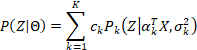(1)

Where Θ = (αk, σk2)T is compound parameter whereas αk and σk2 are regression coefficient and variance of the partial (component) probability distribution Pk(Z|αkTX, σk2). Note, mean of Pk(Z|αkTX, σk2) is αkTX and mixture coefficient isck. In the first approach, regression coefficients αk are estimated by least squares method whereas mixture coefficients ckare estimated by logistic function as follows [11, p. 4]: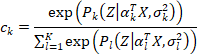(2)

The mixture regression model is: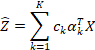(3)

According to the second approach, the joint distribution is defined as follows [12, p. 4]: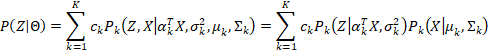(4)

Where αk are regression coefficients and σk2 is variance of the conditional probability distribution Pk(Z|αkTX, σk2) whereas μk and Σk are mean vector and covariance matrix of the prior probability distribution Pk(X|μk, Σk), respectively. The mixture regression model is [12, p. 6]: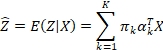(5)

Where,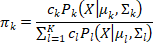(6)

The joint probability can be defined by different way as follows [13, p. 21], [14, p. 24], [15, p. 4]: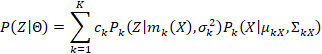(7)

Wheremk(X) and σk2 are mean and variance of Z given the conditional probability distribution Pk(Z|mk(X), σk2) whereas μkX and ΣkX are mean vector and covariance matrix of X given the prior probability distribution Pk(X|μk, Σk). When μkX and ΣkX are calculated from data, other parameters mk(X) and σk2 are estimated for each kth component as follows [13, p. 23], [14, p. 25], [15, p. 5]: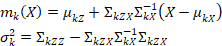(8)

For each kth component, μkZ is sample mean of Z, ΣkZX is vector of covariances of Z and X, and ΣkZZ is sample variance of Z. The mixture regression model becomes [14, p. 25]: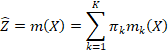(9)

Where,(10)

Grün & Leisch mentioned the full application of mixture model into regression model in which regression coefficients are determined by inverse function of mean of conditional probability distribution as follows: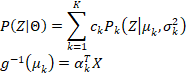(11)

In general, the two approaches in literature do not implement regression mixture model according to EM process in full. They aim to simplify the estimation process in which mixture coefficients ck and regression coefficients αk are estimated one time. Note that EM process is an iterative process in which parameters are improved gradually until convergence. The EM process is slow, but it can balance many factors to reach most optimal parameters. Here we proposed a so-called Mixture Regression Expectation Maximization (MREM) which is the full combination of REM  and mixture model in which we use two EM processes in the same loop. Firstly, we use the first EM process for exponential family of probability distributions to estimate missing values as REM does. Secondly, we use the second EM process to estimate parameters as the full mixture model method does. Anyway, MREM supports fully EM mixture model.

In general, the ideology of combination of regression analysis and mixture model which produces mixture regression is not new, but our proposed MREM is different from other methods in literature because of followings:

• MREM does not use the joint probability distribution. In other words, MREM does not concern the probability distribution of independent variables Xi. MREM does not either use logistic function to estimate mixture coefficients as the first approach does.

• MREM is the full combination of REM  and mixture model in which we use two EM processes in the same loop for estimating missing values and parameters.

• Variance σk2 and regression coefficient αk of the probability Pk(Z|αkTX, σk2) in MREM are estimated and balanced byboth full mixture model and maximum likelihood estimation (MLE). The most similar research to MREM is the weighed least squares algorithm used by Faicel Chamroukhi, Allou Samé, Gérard Govaert, and Patrice Aknin . They firstly split the conditional expectation into two parts at the E-step of EM algorithm and then applied weighed least squares algorithm into the second part for estimate parameters at the M-step [4, pp. 1220-1221].

• Mixture regression models in literature are learned from complete data whereas MREM supports incomplete data.

The methodology of MREM is described in section 2. Section 3 includes experimental results and discussions. Section 4 is the conclusion.

• METHODOLOGY

The probabilistic Mixture Regression Model (MRM) is a combination of normal mixture model and linear regression model. In MRM, the probabilistic Entire Regression Model (ERM) is sum of K weighted probabilistic Partial Regression Models (PRMs). Equation (12) specifies MRM[17, p. 3].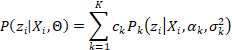(12)

Where,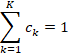Note,Θ is called entire parameter,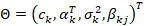The superscript “T” denotes transposition operator in vector and matrix. In equation (12), the probabilistic distribution P(zi|Xi, Θ) represents the ERM where zi is the response variable, dependent variable, or outcome variable. The probabilistic distribution Pk(zi|Xi, αk, σk2) represents the kth PRM zi = αk0 + αk1xi1 + αk2xi2 + … + αknxinwith suppose that each zi conforms to normal distribution according to equation (13) with mean μk = αkTXi and variance σk2.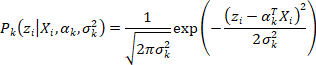(13)

The parameter αk = (αk0, αk1,…, αkn)T is called the kth Partial Regression Coefficient (PRC) and Xi = (1, xi1, xi2,…, xin)T is data vector. Each xij in every PRM is called a regressor, predictor, or independent variable.

In equation (12), each mixture coefficient ck is the prior probability that any zi belongs to the kth PRM. Let Y be random variable representing PRMs, Y = 1, 2,…, K. The mixture coefficient ck is also called the kth weight, which is defined by equation (14). Of course, there are K mixture coefficients, K PRMs, and K PRCs.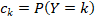(14)

For each kth PRM, suppose each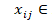​​ has an inverse regression model (IRM) xij = βkj0 + βkj1zi. In other words, xij now is considered as the random variable conforming to normal distribution according to equation (15)[18, p. 8].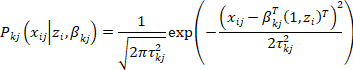(15)

Where βkj = (βkj0, βkj1)T is aninverse regression coefficient (IRC) and (1, zi)T becomes an inverse data vector. The mean and variance of each xij with regard to the inverse distribution Pkj(xij|zi, βkj) are βkjT(1, zi)T and τkj2, respectively. Of course, for each kth PRM, there are nIRMs Pkj(xij|zi, βkj)and n associated IRCs βkj. Totally, there are n*K IRMs associated with n*K IRCs. Suppose IRMs with fixed j have the same mixture model as MRM does. Equation (16) specifies the mixture model of IRMs.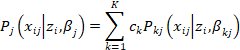(16)

In this research, we focus on estimating the entire parameter Θ = (ck, αk, σk2, βkj)Twhere k is from 1 to K. In other words, we aim to estimate ck, αk, σk2, and βkj for determining the ERM in case of missing data.As a convention, let Θ* = (ck*, αk*, (σk2)*, βkj*)T be the estimate of Θ = (ck, αk, σk2, βkj)T, respectively. Let D = (X, Z) be collected sample in which X is a set of regressors and Z is a set of outcome variables plus values 1,respectively [18, p. 8]with note that both X and Z are incomplete. In other words, X and Z have missing values. As a convention, let ziand xij denote missing values of Z and X, respectively.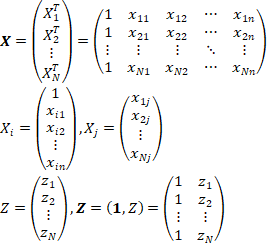(17)

The expectation of sufficient statistic zi regard to the kthPRM Pk(zi|Xi, αk, σk2) is specified by equation (18).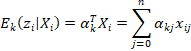(18)

Where xi0=1 for all i. TheexpectationofthesufficientstatisticxijwithregardtoeachIRMPkj(xij|zi, βj)of the kth PRM Pk(zi|Xi, αk, σk2) is specified by equation (19).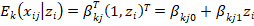(19)

Please pay attention to equations (18) and (19) be cause missing values of data X and data Z will be estimated by these expectation slater.

Because X and Z are incomplete, we apply expectation maximization (EM) algorithm into estimating Θ* = (ck*, αk*, (σk2)*, βkj*)T. According to , EM algorithm has many iterations and each iteration has expectation step (E-step) and maximization step (M-step) for estimating parameters. Given current parameter Θ(t) = (ck(t), αk(t), (σk2)(t), βkj(t))Tat the tth iteration, missing values ziand xij are calculated in E-step so that X and Z become complete. In M-step, the next parameter Θ(t+1) = (ck(t+1), αk(t+1), (σk2)(t+1), βkj(t+1))T is determined based on the complete data X and Z fulfilled in E-step. Here we proposed a so-called Mixture Regression Expectation Maximization (MREM) which is the full combination of Regression Expectation Maximization (REM) algorithm  and mixture model in which we use two EM processes in the same loop. Firstly, we use the first EM process for exponential family of probability distributions to estimate missing values in E-step. The technique is the same to the technique of REM in previous research . Secondly, we use the second EM process to estimate Θ*for full mixture model in M-step.

Firstly, we focus on fulfilling missing values in E-step. The most important problem in our research is how to estimate missing values zi and xij. Recall that, for each kth PRM, every missing value zi is estimated as the expectation based on the current parameter αk(t), according to equation (18).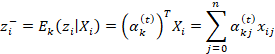Note, xi0 = 1. Let Mi be a set of indices of missing values xij with fixed ifor each kth PRM. In other words, if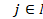​​ then, xij is missing. The set Mi can be empty. The equation (18) is re-written for each kth PRM as follows: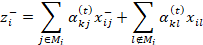According to equation (19),missing value xijis estimated by :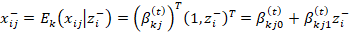Combining equation (18) and equation (19),wehave: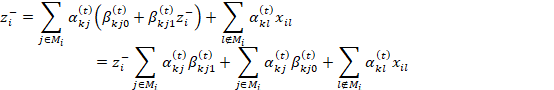It implies :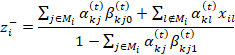As a result, equation (20) is used to estimate or fulfill missing values for each kth PRM.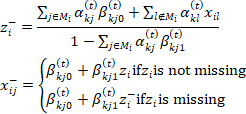(20)

Now in M-step we use EM algorithm again to estimate the next parameter Θ(t+1) = (ck(t+1), αk(t+1), (σk2)(t+1), βkj(t+1))T with current known parameter Θ(t) = (ck(t), αk(t), (σk2)(t), βkj(t+1))T given data X and data Z fulfilled in E-step. The conditional expectation Q(Θ|Θ(t)) with unknown Θ is determined as follows [17, p. 4]: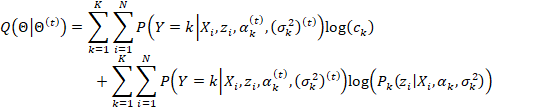The next parameter Θ(t+1) is an constrained optimizer of Q(Θ|Θ(t)). This is the optimization problem.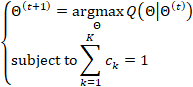By applying Lagrange method, each next mixture coefficient ck(t+1)is specified by equation (21)[17, p. 7].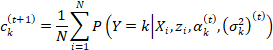(21)

Where P(Y=k | Xi, zi, αk(t), (σk2)(t)) is specified equation (22) [17, p. 3]. It is the conditional probability of the kth PRM given Xi and zi. Please pay attention to this important probability. The proof of equation (22) is found in [17, p. 3], according to Bayes’ rule.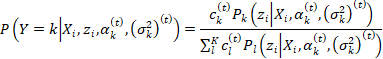(22)

Note, Pk(zi|Xi, αk(t), (σk2)(t)) is determined by equation (13).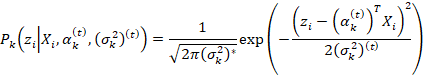By applying Lagrange method, each next regression coefficient αk(t+1)is solution of equation (23) [17, p. 7].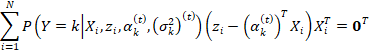(23)

Where 0 = (0, 0,…, 0)T is zero vector and P(Y=k | Xi, zi, αk(t), (σk2)(t)) is specified by equation (22). Equation (23) is equivalent to equation (24):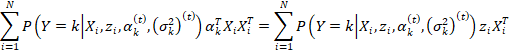(24)

Let,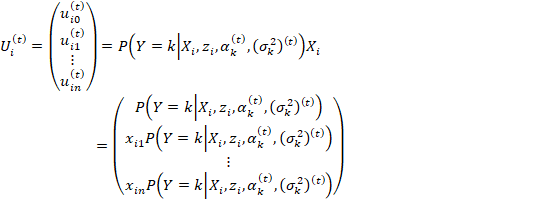Note,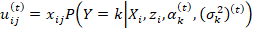The left-hand side of equation (24) becomes: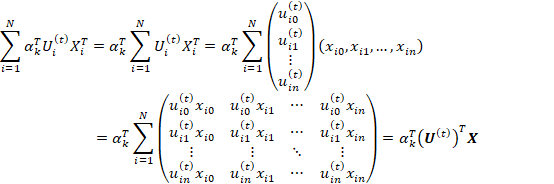Where U(t) is specified by equation (25).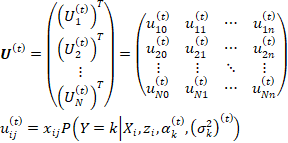(25)

Let,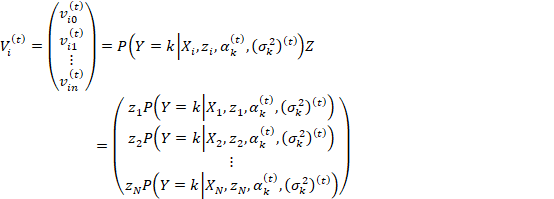Note,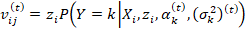The right-hand side of equation (24) becomes: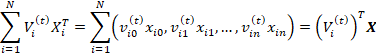Where Vi(t) is specified by equation (26).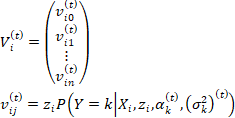(26)

Equation (24) becomes: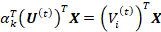Which is equivalent to the following equation: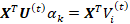As a result, the next regression coefficient αk(t+1), which is solution of equation (23), is specified by equation (27).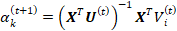(27)

​​ ​​​​ Where X, U(t), and Vi(t) are specified by equations (17), (25), and (26), respectively. The proposed equation (27) is most important in this research because it is the integration of least squares method and mixture model. If we think deeply, it is the key to combine REM and mixture model. In other words, it is the key to combine two EM processes in the same loop.

By applying Lagrange method, each next partial variance (σk2)(t+1) is specified by equation (28) [17, p. 7].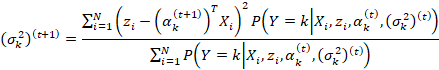(28)

​​ ​​ ​​​​ Where P(Y=k | zi, αk(t), (σk2)(t)) is specified by equation (22) and αk(t+1) is specified by equation (27).The proof of equations (21), (23), and (28) is found in [17, pp. 5-6].

By using maximum likelihood estimation (MLE) method [18, pp. 8-9],weretrieveequation(29)toestimateeach next IRC βkj(t+1)[1, p. 457].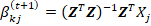(29)

Where Z and Xj are specified in equation (17). Not Z and Xj are fulfilled in E-step. In general, MREM is the full combination of REM and mixture model in which two EM process are applied into the same loop of E-step and M-step. These steps are described in Table 1.

Table1.Mixture Regression Expectation Maximization(MREM)Algorithm.

 E-step: This is the first EM process. Missing values (zi–)kand(xij–)k for each kth PRM are fulfilled by equation (20) given current parameter Θ(t). Please pay attention that each kth PRM owns a partial complete data (Xk, Zk). In other words, the whole sample (X, Z) has K versions (Xk, Zk) for K PRMs. Note, such K versions are changed over each iteration.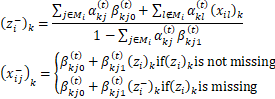The whole sample (X, Z) is fulfilled to become complete data when its missing values zi–andxij– are aggregated from (zi–)k and (xij–)k of K versions (Xk, Zk), by equations (31) and (16).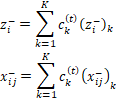M-step: This is the second EM process. The next parameter Θ(t+1) is determined by equations (21),(27), (28), and (29) and the complete data (X, Z) fulfilled in E-step.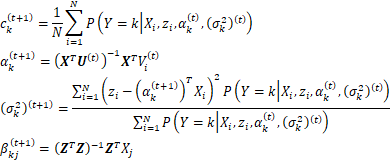WhereU(t) and V(t) are specified by equations (25) and (26) and,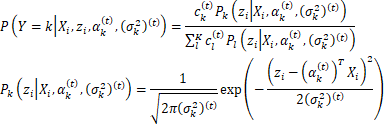The next parameter Θ(t+1) becomes current parameter in the next iteration.

EM algorithm stops if at some tth iteration, we have Θ(t) = Θ(t+1) = Θ*. At that time, Θ* = (ck*, αk*, (σk2)*, βkj*) is the optimal estimate of EM algorithm. Note, Θ(1)at the first iteration is initialized arbitrarily. Here MREM stops if ratio deviation between Θ(t) and Θ(t+1) is smaller than a small enough terminated threshold ε> 0or MREM reaches a large enough number of iterations. The smaller the terminated threshold is, the more accurate MREM is. MREM uses both the terminated threshold ε = 0.1% = 0.001 and the maximum number of iterations (10000). The maximum number of iterations prevents MREM from running for a long time.

MREM is also a clustering method whose each resulted cluster is represented by a pair (αk*, (σk2)*). In other words, each cluster is represented by a PRM. As a convention, these clusters are called conditional clusters or regressive clusters because the mean of each cluster is μk* = (αk*)TXi given a data point Xi. This is an un expecting but interesting result of REM. Given an observation (Xi, zi)T = (xi0, xi1,.., xin, zi)T, if the kth PRM gives out the largest condition probability, it is most likely that Xi belongs to the kth cluster represented by such kth PRM. Let cl(Xi, zi, k) denote the probability of the event that a data point (Xi, zi)T belongs to kth cluster (kth PRM). From equation (22), we have: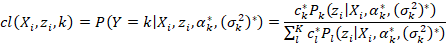We use the complete case method mentioned in [2, p. 3] to improve the convergence of MREM. The parameters (αk(1), βkj(1))T at the first iteration of EM process are initialized in proper way instead that they are initialized in arbitrary way . Let Xk’ be the complete matrix, which is created by removing all rows whose values are missing from Xk. Similarly, let Zk’ be the complete matrix, which is created by removing rows whose weights are missing from Zk. The advanced parameters (αk(1), βkj(1))Tare initialized by equation (30)[1, p. 457].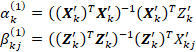(30)

Where Zk’ is the complete vector of non-missing outcome valuesfor each kth PRM and Xkj’ is the complete column vector of non-missing regressor values for each kth PRM.

The evaluation of MREM follows fully mixture model. For example, given input data vector X0 = (x01, x02,…, x0n), let z1, z2,…, zK ​​ be the values evaluated from K PRMs with optimal PRCs αk* resulted from MREM shown in Table 1.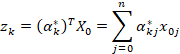Where x00 = 1. The final evaluation z is calculated based on mixture coefficients, given data vector X0 = (x01, x02,…, x0n), as follows: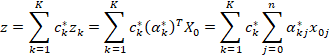(31)

In general, equation (31) is the final regression model of MREM. Following is the proof of equation (31).From equation (12), let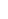be the estimate of response variable z, we have: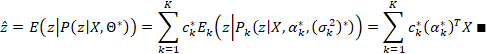We have assumeduntil now that the number K of PRMs is pre-defined and thus, another problem of MREM is how to determine K. Here we propose a so-called increasing algorithm without pre-defining K. In other words, REM associated with increasing algorithm can automatically determine K. Let k be initilized by 1, Followings are two steps of increasing algorithm:

• Executing MREM with k PRMs and then, calculating the fitness f(k) of the resulted mixture model with k PRMs. The fitness f(k) measures adequacy of given mixture model.

• Let l = k + 1, trying to execute MREM with k PRMs and then, to calculate the fitness f(l) of the resulted mixture model with l PRMs. If f(l) >f(k) then, setting k = l and going back step 1; otherwise, the increasing algorithm stops with k PRMs.

The essence of increasing algorithm is how to calculate the fitness f(k) because the final mixture model is the one whose fitness is largest. We define f(k) as the sum of optimal partial probabilities Pc(zc | Xi, αc*, (σc2)*) over all Xi. Equation (32) is the definition of f(k).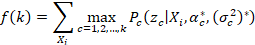(32)

Where,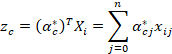For explanation, according equation (32), for each data point Xi, we determine the largest partial probability Pc(zc | Xi, αc*, (σc2)*) over c = 1, 2,…, k as the optimal partial probability. Later one, the fitness f(k) is the sum of all optimal partial probabilities over all Xi. We make experiment on MREM associated with increasing algorithm. I feel that increasing algorithm is not optimal because it seems to be a work-around solution for determining the number K of PRMs but I currently cannot think out better algorithm. In furture, we can research hiearchical clustering or BIC criterion  as alternative solution.

• RESULTS AND DISCUSSIONS

The purpose of the experiment here is to compare MREM and REM. We use ​​ clara sample of R statistical environment for testing MREM and REM. The xclara dataset was edited and published by Vincent Arel-Bundock . It has 3000 points with 3 clusters. There are two numerical variables V1 and V2 as x and y coordinates of points in the xclara dataset. We consider V1 as regressor and V2 as response variable. The xclara dataset was originally used for clustering by Anja Struyf, Mia Hubert, and Peter Rousseeuw  but here it is used for regression analysis.

The dataset is split separately into one training dataset (50% sample) and one testing dataset (50% sample). Later on, the training dataset is made sparse with loss ratios 10%, 20%, 30%, 40%, 50%, 60%, 70%, 80%, and 90%, which is similar to our previous research . Missing values are made randomly regardless of regressors or response variable. For example, the xclar atraining dataset (50% xclar asample) has 50%*3000=1500 rows and each row has 2 columns (V1 and V2) and so the training dataset has 1500*5 = 7500 cells. If loss ratio is 10%, there are only 10%*7500=750 missing values which are made randomly among such 7500 cells. In other words, the incomplete training dataset with loss ratio 10% has 7500 – 750 = 6750 non-missing values. Of course, the testing dataset (50% sample) is not made sparse. Each pair of incomplete training dataset and testing dataset is called testing pair. There are ten testing pairs for each sample. As a convention, the origin testing pair which has no missing value in training dataset is the 0th pair. The 0th pair is called complete pair whereas the 1st, 2nd, 3rd, 4th, 5th, 6th, 7th, 8th, and 9th pairs are called incomplete pairs.

Firstly, we test MREM and REM with xclara sample. Table 2 shows ten testing pairs of ​​ xclara sample.

Table 2. Ten testing pairs of gestational sample.

 Pair Training dataset Testing dataset Loss ratio 0 xclara.base xclara.test 0% 1 xclara.base.0.1.miss xclara.test 10% 2 xclara.base.0.2.miss xclara.test 20% 3 xclara.base.0.3.miss xclara.test 30% 4 xclara.base.0.4.miss xclara.test 40% 5 xclara.base.0.5.miss xclara.test 50% 6 xclara.base.0.6.miss xclara.test 60% 7 xclara.base.0.7.miss xclara.test 70% 8 xclara.base.0.8.miss xclara.test 80% 9 xclara.base.0.9.miss xclara.test 90%

Table 3 shows ten resulted regression models of REM corresponding to ten testing pairs of ​​ xclara sample.

Table 3. Ten resulted regression models of REM given xclara sample.

 Pair Regression model 0 V2 = 34.0445 - 0.2790*(V1) 1 V2 = 36.8255 - 0.3384*(V1) 2 V2 = 36.5624 - 0.3393*(V1) 3 V2 = 37.4537 - 0.4022*(V1) 4 V2 = 45.8814 - 0.5830*(V1) 5 V2 = 48.8888 - 0.6477*(V1) 6 V2 = 55.9764 - 0.8593*(V1) 7 V2 = 48.8888 - 0.6477*(V1) 8 V2 = 69.1886 - 1.0823*(V1) 9 V2 = 62.2939 - 1.1417*(V1)

Table 4shows ten resulted mixture regression models of MREM corresponding to ten testing pairs of ​​ xclara sample.

Table 4. Ten resulted mixture regression models of MREM given xclara sample.

 Pair Mixture regression model 0 {V2 = 34.0445 - 0.2790*(V1): coeff=1.0000, var=962.0000} 1 {V2 = 16.6425 - 0.3065*(V1): coeff=0.6654, var=188.4319}, {V2 = 62.3919 - 0.0429*(V1): coeff=0.3346, var=86.8709} 2 {V2 = 13.2805 - 0.3332*(V1): coeff=0.4909, var=124.9130}, {V2 = 64.0639 - 0.0980*(V1): coeff=0.3031, var=102.6651}, {V2 = 32.5172 - 0.2432*(V1): coeff=0.2060, var=0.0573} 3 {V2 = 13.2047 - 0.3220*(V1): coeff=0.4410, var=138.2844}, {V2 = 66.5083 - 0.1668*(V1): coeff=0.2424, var=91.6464}, {V2 = 31.9337 - 0.2667*(V1): coeff=0.3166, var=0.0323} 4 {V2 = 14.5836 - 0.3404*(V1): coeff=0.3772, var=132.5547}, {V2 = 65.9884 - 0.1683*(V1): coeff=0.2224, var=99.6319}, {V2 = 33.3280 - 0.2766*(V1): coeff=0.4004, var=0.0547} 5 {V2 = 33.5698 - 0.2666*(V1): coeff=0.5096, var=0.0346}, {V2 = 65.8616 - 0.1536*(V1): coeff=0.1906, var=83.6705}, {V2 = 13.6946 - 0.3393*(V1): coeff=0.2998, var=152.4883} 6 {V2 = 73.1729 - 1.1518*(V1): coeff=0.8835, var=49.5093}, {V2 = 7.3758 + 1.0052*(V1): coeff=0.1165, var=296.8865} 7 {V2 = 33.5698 - 0.2666*(V1): coeff=0.5096, var=0.0346}, {V2 = 65.8616 - 0.1536*(V1): coeff=0.1906, var=83.6705}, {V2 = 13.6946 - 0.3393*(V1): coeff=0.2998, var=152.4883} 8 {V2 = 69.1886 - 1.0823*(V1): coeff=1.0000, var=58.6193} 9 {V2 = 62.2939 - 1.1417*(V1): coeff=1.0000, var=21.6927}

In Table 4, each PRM is wrapped in two brackets “{.}”. Notation “coeff” denotes mixture coefficient and notation “var” denotes the variance of a PRM. Note, MREM is also a clustering method where each regressive cluster is represented by a PRM. In other words, each PRM is considered as a regressive mean or regressive representative of a regressive cluster. However, regressive clustering with MREM is different from usual clustering. When data is visualized, we will see that the good numbers of regressive clusters is 2 whereas the best number of usual clusters in xclara sample is 3 . Figure 1 shows the unique regressive cluster of the training dataset of the 0th testing pair.Figure 1. Unique cluster of the training dataset of the 0th pair.

The PRM is drawn as a thin and solid line going through theunique regressive cluster. Of course, such solid line shows the line equation of the PRM, V2 = 34.0445 - 0.2790*(V1).

Figure 2 shows two regressive clusters of the training dataset of the 1st testing pair. Note, missing values in the 1st training dataset are fulfilled by after MREM finished.Figure 2. Two regressive clusters of the training dataset of the 1st pair.

As seen in figure 2, there are two solid lines which represents two PRMs. The upper solid line represents the PRM V2 = 64.0639 - 0.0980*(V1) whereas the lower solid line represents the PRMV2 = 62.3919 - 0.0429*(V1).

Given xclara sample, we compare MREM with REM with regard to the ratio mean absolute error (RMAE). Let W = {w1, w2,…, wK} and V = {v1, v2,…, vK} be sets of actual weights and estimated weights, respectively. Equation (33) specifies the RMAE metric[23, p. 814].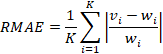(33)

The smaller the RMAE is, the more accurate the algorithm is. Table 5is the comparison of REM and MREM with regard to RMAE given xclara sample.

Table 5. Comparison of REM and MREM regarding RMAE, given xclara sample

 Pair RMAE(REM) RMAE(MREM) 0 5.4722 5.4722 1 5.3672 5.7804 2 5.2846 5.6044 3 4.6337 5.1166 4 4.3681 5.3686 5 4.3025 5.5701 8 4.912 5.2689 7 4.3025 5.5701 8 6.1709 6.1709 9 7.2932 7.2932 Average 5.2107 5.7215

From Table 5, given xclara sample, MREM is less accurate than REM according RMAE metric. When I test MREM with other samples, it is also not better than REM in accuracy. This is an unexpected result which is easy to lead a conclusion that MREM is not useful. The reason of this unexpected result is that we cannot choose a right regressive cluster for given regressors X to estimate response value z. The equation (31) is the average formula for evaluating mixture model orver K PRMs and so it will produce unexpected bias. For example, I generate a sample in which there is only one regressor x and only one response variable z. There are 1000 points (x, z) in the generated sample. The variable x is randomized from 0 to 1. From 0 to 0.5, x and z satisfy the linear equation z = x with variance 0.001. From 0.5 to 1, x and z satisfy the linear equation z = 1 – x with variance 0.001. The probability of the equation z = x is equal to the probability of the equation z = 1 – x, which is 0.5. MREM with pre-defined K = 2 produces the mixture model {{z = 0.0036 + 0.9765*(x), coeff=0.4634, var=0.0011}, {z = 0.9767 - 0.9713*(x), coeff=0.5366, var=0.0009}} which is an approximation of such two linear equations. Without loss of generity, training dataset is also used as testing dataset. Figure 3 shows the mixture model (K = 2) with regressive clusters.Figure 3. Regressive clusters of the generated sample.

In figure 3, the approximation of the equation z = xis z = 0.0036 + 0.9765*(x)with mixture coefficient c1 = 0.4634whereas the approximation of the equation z = 1 – xis z = 0.9767 - 0.9713*(x)with mixture coefficient c2 = 0.5366. Given generated sample, the RMAE of MREM is 5.3957which is worse than the RMAE of REM (2.5790). Obviously, although MREM produces a good approximation of the linear equations z = x and z = 1 – x such as z = 0.0036 + 0.9765*(x) and z = 0.9767 - 0.9713*(x), respectively but it cannot select the right one for estimating response values. MREM instead produces average values according equation (31). As a result, MREM gives out worse accuracy. If MREM can select the equation z = 0.0036 + 0.9765*(x)and the equation z = 0.9767 - 0.9713*(x)for estimating response values for 0 ≤x< 0.5 and 0.5 ≤x≤ 1, respectively then, the RMAE of MREM becomes 0.4 which is better the RMAE of REM (2.5790). In general, MREM is still useful because a different approach for mixture model can be referred by fusing linear equations ofMREM into a unique curve equation. The curve modeling with regression analysis was proposed by FaicelChamroukhi, AllouSamé, Gérard Govaert, and Patrice Aknin.

• CONCLUSIONS

In general, the essence of MREM is to integrate two EM processes (one for exponential estimation of missing values and one for mixture model estimation of parameters) into the same loop with expectation that MREM will take advantages of both REM in fulfilling incomplete data and mixture model in processing complicatedly varied data. The proposed equation (27) is the key to combine REM and mixture model. Unfortunately, experimental result shows that MREM is less accurate than REM because MREM causes biases in estimating response values by average formula specified by equation (31). However, MREM is essential because for further research, we will research some approximation techniques to fuse linear equations of mixture model into a unique curve equation.The curve modeling with regression analysis was proposed by Faicel Chamroukhi, AllouSamé, Gérard Govaert, and Patrice Aknin.

• CONFLICTS OF INTEREST

The authors declare that there is no conflict of interest regarding the publication of this article.

• ACKNOWLEDGMENTS

We express our deep gratitude to Prof. Dr. Thu-Hang Thi Ho (Vinh Long General Hospital – Vietnam) and Prof. Bich-Ngoc Tran who gave us helpful works, helpful comments, and testing sample to develop and test MREM with note that MREM is derived from REM.

• REFERENCES

• Montgomery, D. C.; Runger, G. C. Applied Statistics and Probability for Engineers, 5th ed.; John Wiley & Sons: Hoboken, New Jersey, USA, 2010; p. 792.Available online: https://books.google.com.vn/books?id=_f4KrEcNAfEC (accessed on 6th September 2016).

• Horton, N. J.; Kleinman, K. P. Much ado about nothing: A comparison of missing data methods and software to fit incomplete data regression models. The American Statistician, February 2007, vol. 61, no. 1, pp. 79-90. DOI:10.1198/000313007X172556.

• Nguyen, L.;Ho, T.-H. T. Fetal Weight Estimation in Case of Missing Data. Experimental Medicine (EM) - Special Issue “Medicine and Healthy Food”, 2018.

• Chamroukhi, F.;Samé, A.;Govaert, G.;Aknin, P. (2010, March). A hidden process regression model for functional data description: Application to curve discrimination. (Wang, Z.; Hoi, S.; Eds.) Neurocomputing, March 2010, 73(7-9), 1210-1221,DOI:10.1016/j.neucom.2009.12.023. Available online:https://www.sciencedirect.com/science/article/pii/S0925231210000287 (accessed on 12ndOctober 2018).

• Kokic, P.TheEMAlgorithmforaMultivariateRegressionModel:includingitsapplicationstoanon-parametricregressionmodelandamultivariatetimeseriesmodel.QantarisGmbH,Frankfurt,2002.Available online: https://www.cs.york.ac.uk/euredit/_temp/The%20Euredit%20Software/NAG%20Prototype%20platform/WorkingPaper4.pdf (accessed on 30th June 2018).

• Ghitany, M. E.;Karlis, D.;Al-Mutairi, D. K.;Al-Awadhi, F.AnEMAlgorithmforMultivariateMixedPoissonRegressionModelsanditsApplication.AppliedMathematicalSciences, 2012,vol.6,no.137,pp.6843-6856.Available online: http://www.m-hikari.com/ams/ams-2012/ams-137-140-2012/ghitanyAMS137-140-2012.pdf(accessed on 3rd July2018).

• Anderson, B.;Hardin, M. J.ModifiedlogisticregressionusingtheEMalgorithmforrejectinference.InternationalJournalofDataAnalysisTechniquesandStrategies, 1st January 2013,vol.5,no.4,pp.359-373. DOI:10.1504/IJDATS.2013.058582.

• Zhang, X.;Deng, J.;Su, R.TheEMalgorithmforalinearregressionmodelwithapplicationtoadiabetesdata.InProceedings of the2016InternationalConferenceonProgressinInformaticsandComputing(PIC),Shanghai,China, 2016.DOI:10.1109/PIC.2016.7949477.

• Haitovsky, Y.MissingDatainRegressionAnalysis.JournaloftheRoyalStatisticalSociety:SeriesB(Methodological), 1st January 1968,vol.30,no.1,pp.67-82.Available online: https://www.jstor.org/stable/2984459(accessed on 3rdJuly2018).

• Robins, J. M.;Rotnitzki, A.;Zhao, L. P.AnalysisofSemiparametricRegressionModelsforRepeatedOutcomesinthePresenceofMissingData.JournaloftheAmericanStatisticalAssociation, March 1995,vol.90,no.429,pp.106-121.DOI:10.2307/2291134.

• Lamont, A. E.; Vermunt, J. K.; Lee, V. H. M.Regression mixture models: Does modeling the covariance between independent variables and latent classes improve the results? Multivariate Behavioral Research, January 2016, vol. 51, no. 1, pp. 35-52. DOI:10.1080/00273171.2015.1095063.

• Hoshikawa, T. Mixture regression for observational data, with application to functional regression models.arXiv preprint, 30th June 2013.arXiv:1307.0170.

• Nguyen, H. D. Finite Mixture Models for Regression Problems. The University of Queensland, Brisbane, 2015.DOI:10.14264/uql.2015.584.

• Sung, H. G.Gaussian Mixture Regression and Classification. Rice University, Houston, 2004.Available online: https://scholarship.rice.edu/handle/1911/18710(accessed on 4thSeptember2018).

• Tian, Y.; Sigal, L.; Badino, H.; Torre, F. D. l.; Liu, Y. Latent Gaussian Mixture Regression for Human Pose Estimation.InLecture Notes in Computer Science, vol 6494, Proceedings of The 10th Asian Conference on Computer Vision (ACCV 2010), Queenstown, 2010. DOI:10.1007/978-3-642-19318-7_53.

• Grün, B.; Leisch, F. Finite Mixtures of Generalized Linear Regression Models. University of Munich, Munich, 2007.Available online: https://pdfs.semanticscholar.org/e0d5/6ac54b80a1a4e274f11b1d86840461cc542c.pdf(accessed on 4thSeptember2018).

• Bilmes, J. A. A Gentle Tutorial of the EM Algorithm and its Application to Parameter Estimation for Gaussian Mixture and Hidden Markov Models. University of Washington, Berkeley, 1998.Available online: http://melodi.ee.washington.edu/people/bilmes/mypubs/bilmes1997-em.pdf(accessed on 17th September 2013).

• Lindsten, F.;Schön, T. B.;Svensson, A.;Wahlström, N.Probabilisticmodeling–linearregression&Gaussianprocesses. UppsalaUniversity,Uppsala,2017.Available online: http://www.it.uu.se/edu/course/homepage/sml/literature/probabilistic_modeling_compendium.pdf (accessed on 24th January 2018).

• Dempster, A. P.;Laird, N. M.;Rubin, D. B.MaximumLikelihoodfromIncompleteDataviatheEMAlgorithm.JournaloftheRoyalStatisticalSociety,SeriesB(Methodological), 1977,vol.39,no.1,pp.1-38.

• Nguyen, L.;Ho, T.-H. T. Early Fetal Weight Estimation with Expectation Maximization Algorithm. Experimental Medicine (EM), 2018, 1(1), 12-30.DOI:10.31058/j.em.2018.11002.

• Arel-Bundock, V. (2018, June 28). Rdatasets - An archive of datasets distributed with R. GitHub, 28th June 2018.Available online: http://vincentarelbundock.github.io/Rdatasets/csv/cluster/xclara.csv(accessed on 11stSeptember2018).

• Struyf, A.; Hubert, M.;Rousseeuw, P. J. (1996). Clustering in an Object-Oriented Environment. Journal of Statistical Software, 1996, 1(4), 1-30,DOI:10.18637/jss.v001.i04.Available online: http://www.jstatsoft.org/v01/i04(accessed on 10thOctober2018).

• Pinette, M. G.; Pan, Y.; Pinette, S. G.; Blackstone, J.; Garrett, J.; Cartin, A. Estimation of Fetal Weight: Mean Value from Multiple Formulas. Journal of Ultrasound in Medicine, 1st December 1999, vol. 18, no. 12, pp. 813-817. Available online: https://www.ncbi.nlm.nih.gov/pubmed/10591444(accessed on 9thOctober2016).